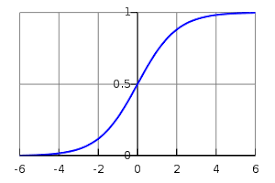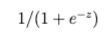# A Beginner's Guide to Logistic Regression For Machine Learning

Logistic regression converts input data into one of two categories. Show it portraits and it will categorize them as male or female. It functions as a binomial classifier.

You can think of logistic regression as an on-off switch. It can stand alone, or some version of it may be used as a mathematical component to form switches, or gates, that relay or block the flow of information.

Like any switch, logistic regression can be a component in a larger circuit. Logistic regression is the transistor of machine learning, the switch upon which larger and more universal computation engines are built.

Instead of regulating current, or voltage flow, in a circuit board, logistic regression regulates the signal flowing from input data through a larger algorithm to the predictions that it makes.

On a circuit board, a transistor might receive voltage that opens a current to turn on a light. In a machine-learning algorithm, logistic regression allows signal through, or not, to make a classification. Hot dog or not hot dog.The image above traces a logistic function. As you can see, it is s-shaped, or sigmoid, flattening out at the top and bottom, while transitioning quickly between the two states before entering one of the long, asymptotic tails. What that means is, input can build up for a long time while still being interpreted by the function as “off”, but by adding incrementally more signal at just the right place, the function flips to “on”, and it remains “on” forever.

Logistic regression is widely used in statistics, and it was originally applied in ecology to the study of populations, whose growth tends to plateau as they exhaust the resources at their disposal.1

As a function, logistic regression is simply an S-shaped curve that can ingest any real-valued number, and translate it to a value between 0 and 1. In the graph above, we take continuous values between -6 and 6 and map them to values between 0 an 1. Here is the formula that performs that mapping:Fwiw, `e` is a mathematical constant known as Euler’s number, an irrational number that is approximately 2.71828. It is the base of the natural logarithms (which answer the question: which number x, when multiplied by itself, produces number y. Logarithms look like a flattening hill, while exponential functions, their inverse, look like a mountain being beamed up to a space ship).

In this same formula, `z` is the sum of all inputs that are being used to make a prediction; i.e. `z = b0 + b1 + b2 + b3 ...`

## Neural Networks and Logistic Regression

Broadly speaking, neural networks are used for the purpose of clustering through unsupervised learning, classification through supervised learning, or regression. That is, they help group unlabeled data, categorize labeled data or predict continuous values.

Classifiers typically use a form of logistic regression in the net’s final layer to convert continuous data into dummy variables like 0 and 1 – e.g. given someone’s height, weight and age you might bucket them as a heart-disease candidate or not.

### Working on a New AI Startup?

Find Out How Page One Can Support You

## Logistic Regression Predicts Probabilities

When you map all your inputs to a value between 0 and 1, one way to think about the result is as a probability. Since logistic regression is a binomial classifier, bucketing everything it sees as either `hotdog` or `not_hotdog`, then it’s results can be interpreted as the likelihood that the data in question is a hot dog. Or spam. Or fraud. The main category is known as the default class, usually the represented as the 1 in the classifier.

You can express logistic regression in a Bayesian way:

`P(food=hotdog|color)`

While you may be using logistic regression as a classifier for hot dogs, the output it’s really giving you is the probability that the data in question is a hot dog.